# Equivalent Inductance of Series and Parallel Inductors (With Mutual Inductance)

Contents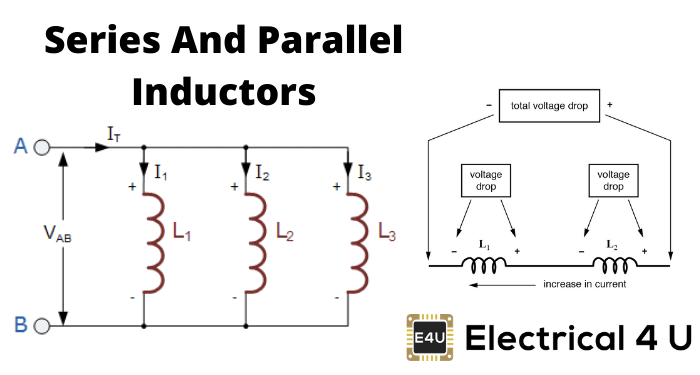When inductors are connected in series, the equivalent inductance of the combination will be the sum of all individual inductors’ inductance. This is just like the equivalent resistance of series-connected resistors.

But in the case of inductors, we sometimes have to consider the effect of mutual inductance between the inductors.

Then, to calculate the inductance of each inductor, we consider both the self-inductance and mutual inductance of the inductor.

The mutual inductance will be either added or subtracted from the self-inductance depending on magnetically coupled inductors’ polarity.

Now, without considering mutual inductances, we can write the equivalent inductance of series-connected inductors as,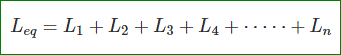When inductors are connected in parallel, the reciprocal of the equivalent inductance of the combination will be the sum of individual inductances’ reciprocal.

This is just like the equivalent resistance of parallel-connected resistors. We may also have to consider the effect of mutual inductance in the same way if required.

We will learn the effect of mutual inductance on parallel inductors later in this article. Without considering the effect of mutual inductance, we can write,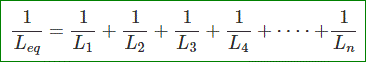An inductor is a passive circuit element. Let us find out the equivalent inductance of series-connected and parallel-connected inductors.

Let us consider n number of inductors connected in series as shown below.Let us also consider that,
the inductance of inductor 1 and voltage drop across it is L1 and v1, respectively,
the inductance of inductor 1 and voltage drop across it is L2 and v2, respectively,
the inductance of inductor 1 and voltage drop across it is L3 and v3, respectively,
the inductance of inductor 1 and voltage drop across it is L4 and v4, respectively,
the inductance of inductor 1 and voltage drop across it are Ln and vn, respectively.

Now, applying Kirchhoff’s Voltage Law, we get, total voltage drop (v) across the series combination of the inductors,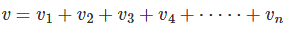The voltage drop across an inductor of inductance L can be expressed as,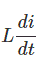Where i is the instantaneous current through the inductor.

As all inductors of the combinations are connected in series, the current through each of the inductors is the same, and say it is also i. So, from the above KVL equation, we get,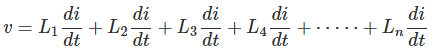This equation can be rewritten as,Where Leq is the equivalent inductance of the series combined inductors. Hence,The equivalent inductance of series-connected inductors is simply the arithmetic sum of the inductance of individual inductors.

Let us consider n number of inductors connected in parallel, as shown below.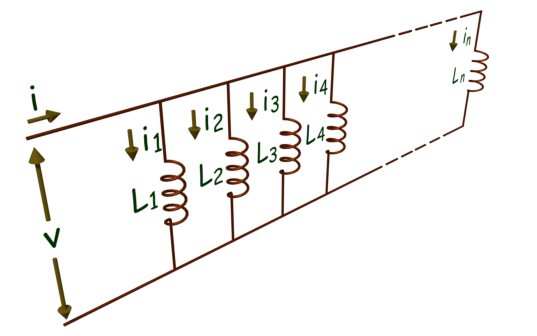Let us also consider that,
the inductance of inductor 1 and current through it is L1 and i1, respectively,
the inductance of inductor 1 and current through it is L2 and i2, respectively,
the inductance of inductor 1 and current through it is L3 and i3, respectively,
the inductance of inductor 1 and current through it is L4 and i4, respectively,
the inductance of inductor 1 and current through it is Ln and in, respectively.

Now, applying Kirchhoff’s Current Law, we get total current (i) entering in the parallel combination of the inductors,The current through an inductor of inductance L can be expressed as,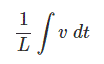Where v is the instantaneous voltage across the inductor.

As all inductors of the combinations are connected in parallel, here, the voltage drop across each of the inductors is the same, and say also it is v. So, from the above KCL equation, we get,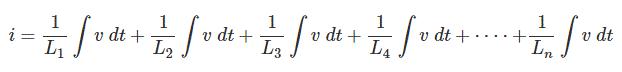This equation can be rewritten as,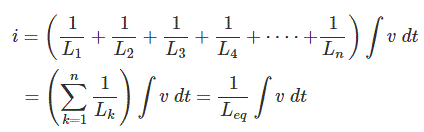Where Leq is the equivalent inductance of the parallel combined inductors. Hence,Reciprocal of the equivalent inductance of parallel-connected inductors is simply an arithmetic sum of the reciprocal of individual inductors’ inductance.

## Effect of Mutual Induction in Series Connected Inductors

Whenever more than one inductors come in closer, there may be mutual induction between them. If more than one inductor is connected in series and the flux of one inductor links the other, we have to consider mutual inductance during equivalent inductance calculations.

For this purpose, we use the dot convention. Here each of the inductors is marked with a dot at one end.

The current entering through the dotted terminal of one inductor will induce a voltage in the other inductor with positive polarity at the latter’s dotted terminal. Let us consider the following example.As inductors are in series same current will flow to these inductors.

Hence when the current enters through the dotted terminal of inductor 1, the current enters through the dotted terminal of inductor 2.

Inductor 2 will induce the voltage across inductor 1 with positive polarity at the dotted end of inductor 1.

Current enters through the dotted terminal of inductor 1 will induce the voltage across inductor 2 with positive polarity at the dotted end of inductor 2.

As both mutually induced EMFs are in the direction of self-induced emf the equivalent impedances, the mutual inductance will be added to self-inductance to calculate equivalent impedance.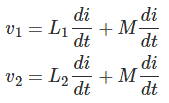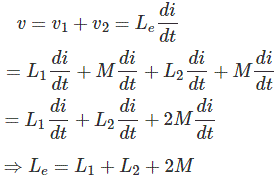Here in this second example, as per dot convention provided in the figure below, the current is entering through the dotted terminal of one inductor, and the same current is leaving the dotted terminal of the other inductor.

In that case polarity of mutually induced emf differs from the self-induced emf. The equivalent inductance of the combination will be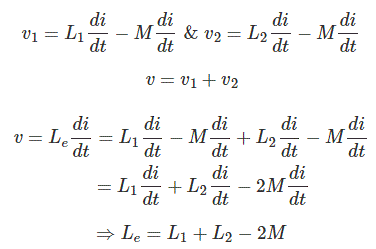## Effect of Mutual Induction in Parallel Connected Inductors

Now we will come to the effect of mutual inductance in parallel connected inductors. Here in this example, dots are given to the same side of two inductors.

When current is entering through the dotted terminal of inductor 1, the emf induced has the positive polarity at the dotted end of inductor 2.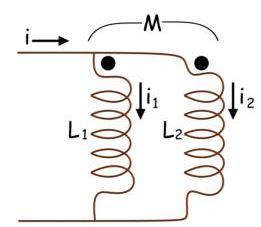Similarly, when current enters through the dotted terminal of inductor 2, the emf induced in inductor 1 has positive polarity at the dotted end of inductor 1. So equivalent inductance is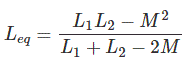Similarly, when two parallel inductors are dotted in the following manner, the equivalent inductance will be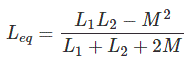Want To Learn Faster? 🎓
Get electrical articles delivered to your inbox every week.
No credit card required—it’s 100% free.